Common Core: 2nd Grade Math : Number Names for Hundreds: CCSS.Math.Content.2.NBT.A.1b

Example Questions

← Previous 1 3 4

Example Question #1 : Number Names For Hundreds: Ccss.Math.Content.2.Nbt.A.1b

How many ones are in the number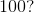Three

Two

Zero

Four

One

Zero

Explanation:

There is ain the ones place which means there are no ones in the number.

Example Question #2 : Number Names For Hundreds: Ccss.Math.Content.2.Nbt.A.1b

How many tens are in the number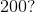Three

Four

Zero

Two

One

Zero

Explanation:

There is ain the tens place which means there are no tens in the number.

Example Question #3 : Number Names For Hundreds: Ccss.Math.Content.2.Nbt.A.1b

How many hundreds are in the number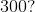Thirteen

Three hundred

Three

Thirty

Zero

Three

Explanation:

There is ain the hundreds place which means there are three hundreds in the number.

Example Question #4 : Number Names For Hundreds: Ccss.Math.Content.2.Nbt.A.1b

How many ones are in the number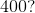Forty

Two

Zero

Three

Four

Zero

Explanation:

There is ain the ones place which means there are no ones in the number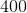.

Example Question #5 : Number Names For Hundreds: Ccss.Math.Content.2.Nbt.A.1b

How many tens are in the number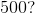Five

Three

One

Zero

Six

Zero

Explanation:

There is ain the tens place which means there are no tens in the number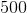.

Example Question #6 : Number Names For Hundreds: Ccss.Math.Content.2.Nbt.A.1b

How many hundreds are in the number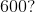Sixty

Six

Nine

Six hundred

Three

Six

Explanation:

There is ain the hundreds place which means there are six hundreds in the number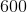.

Example Question #7 : Number Names For Hundreds: Ccss.Math.Content.2.Nbt.A.1b

How many ones are in the number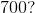Seven

Seventy-seven

Zero

Fourteen

Seventy

Zero

Explanation:

There is ain the ones place which means there are no ones in the number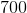.

Example Question #8 : Number Names For Hundreds: Ccss.Math.Content.2.Nbt.A.1b

How many tens are in the number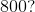Zero

Sixty

Eight hundred

Eight

Eighteen

Zero

Explanation:

There is ain the tens place which means there are no tens in the number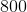.

Example Question #9 : Number Names For Hundreds: Ccss.Math.Content.2.Nbt.A.1b

How many hundreds are in the number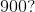Nine

Ninteen

Nine hundred

Ninty

Ninty-nine

Nine

Explanation:

There is ain the hundreds place which means there are nine hundreds in the number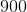.

Example Question #10 : Number Names For Hundreds: Ccss.Math.Content.2.Nbt.A.1b

How many hundreds are in the number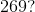Twelve

Two

Nine

Six

Twenty

There is ain the hundreds place which means there are two hundreds in the number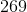.